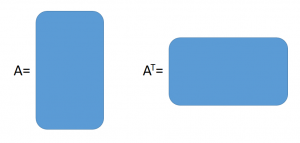16
Feb 16

## OLS estimator for multiple regression - simplified derivationOLS estimator for multiple regression - as simple as possible

Here I try to explain a couple of ideas to folks not familiar with (or afraid of?) matrix algebra.

A matrix is a rectangular table of numbers. Operations with them most of the time are performed like with numbers. For example, for numbers we know that$a+b=b+a$. For matrices this is also true, except that they often are denoted with capital letters:$A+B=B+A$. It is easier to describe differences than similarities.

(1) One of the differences is that for matrices we can define a new operation called transposition: the columns of the original matrix$A$ are put into rows of a new matrix, which is called a transposed of$A$. Visualize it like this: if$A$ has more rows than columns, then for the transposed the opposite will be true:(2) We know that the number$1$ has the property that$1\times a=a$. For matrices, the analog is$I\times A=A$ where$I$ is a special matrix called identity.

(3) The property$\frac{1}{a}a=1$ we have for nonzero numbers generalizes for matrices except that instead of$\frac{1}{A}$ we write$A^{-1}$. Thus, the inverse matrix has the property that$A^{-1}A=I$.

(4) You don't need to worry about how these operations are performed when you are given specific numerical matrices, because they can be easily done in Excel. All you have to do is watch that theoretical requirements are not violated. One of them is that, in general, matrices in a product cannot change places:$AB\ne BA$.

Here is an example that continues my previous post about simplified derivation of the OLS estimator. Consider multiple regression

(5)$y=X\beta+u$

where$y$ is the dependent variable,$X$ is an independent variable,$\beta$ is the parameter to estimate and$u$ is the error. Multiplying from the left equation (5) by$X^T$ we get$X^Ty=X^TX\beta+X^Tu$. As in my previous post, we get rid of the term containing the error by formally putting$X^Tu=0$. The resulting equation$X^Ty=X^TX\beta$ we solve for$\beta$ by multiplying by$(X^TX)^{-1}$ from the left:$(X^TX)^{-1}X^Ty=(X^TX)^{-1}(X^TX)\beta=(using\ (3))=I\beta=(using\ (2))=\beta.$

Putting the hat on$\beta$, we arrive to the OLS estimator for multiple regression$\hat{\beta}=(X^TX)^{-1}X^Ty$. Like in the previous post, the whole derivation takes just one paragraph!

Caveat. See the rigorous derivation here. My objective is not rigor but to give you something easy to do and remember.